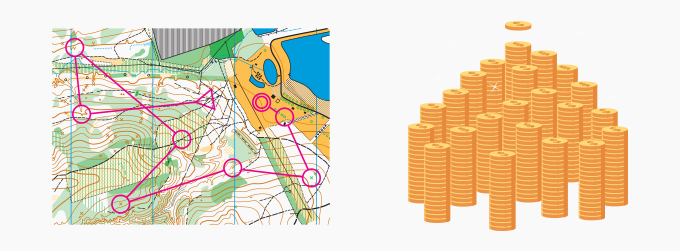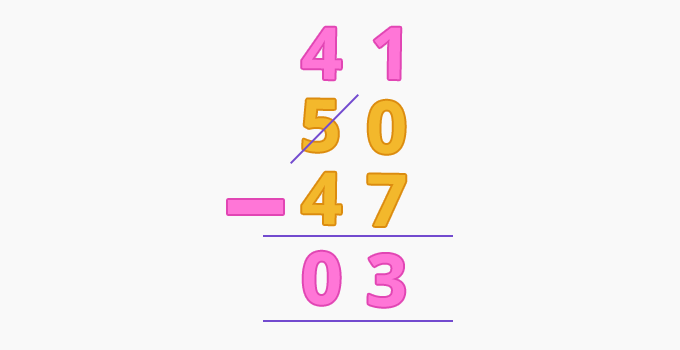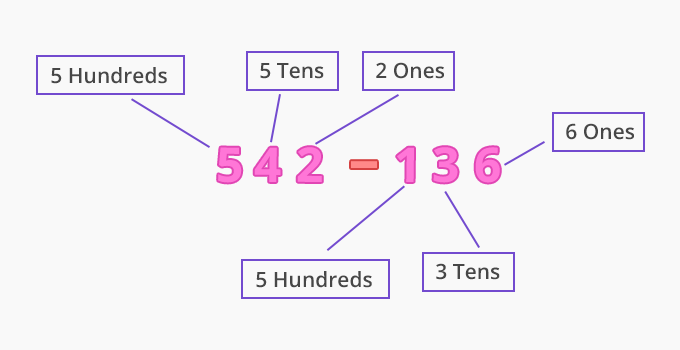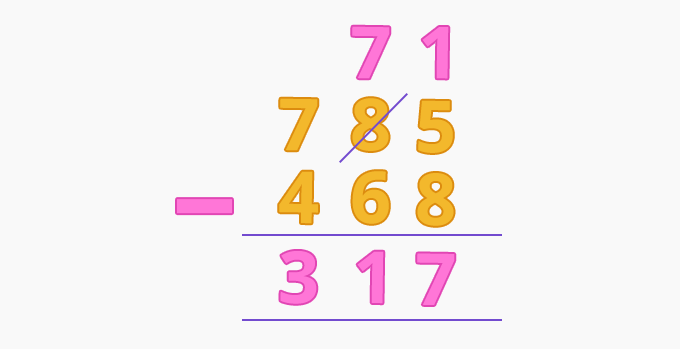# Subtraction With Regrouping - Definition with Examples

The Complete K-5 Math Learning Program Built for Your Child

• 40 Million Kids

Loved by kids and parent worldwide

• 50,000 Schools

Trusted by teachers across schools

• Comprehensive Curriculum

Aligned to Common Core

## Subtraction with Regrouping

Regrouping in subtraction is a process of exchanging one tens into ten ones. We use regrouping in subtraction when the minuend is smaller than the subtrahend.

Uses of subtraction with regrouping

Subtraction with regrouping is very useful in our daily life. Some examples are given below:

1. To measure distance

2. To deal with money

3. To measure timeUsing subtraction with regrouping

We use subtraction with regrouping to work out different subtraction problems.

For example, Ray buys chocolates worth \$47. He pays by giving a \$50 bill. How much money will he get back?

Ray pays \$50, means 5 tens and 0 ones. He bought chocolates worth \$47, means 4 tens and 7 ones. Here we can see the minuend (0) is smaller than the subtrahend (7). We can regroup them by borrowing 1 ten to make ten ones.Ray has \$3 left with him.

Example 2: 542 – 136Example 2: In a school, there are 785 students, and  468 of them are girls. Find the number of boys in the school?

Total number of students = 785

Number of girls in the school = 468

Number of boys in the school = 785 – 468 = 317Fun Facts We can always check our answer by adding the subtrahend and the difference to get the minuend as addition is the inverse of subtraction.

Won Numerous Awards & Honors